## Convergence of Power Series

Theorem 6.5.6   Given a power series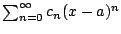, there are exactly three possibilities:
1. The series conveges only when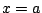.
2. The series conveges for all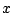.
3. There is an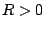(called the radius of convergence'') such thatconverges for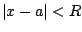and diverges for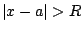.

Example 6.5.7   For the power series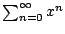, the radius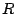of convergence is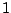.

Definition 6.5.8 (Radius of Convergence)   As mentioned in the theorem,is called the radius of convergence.

If the series converges only at, we say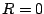, and if the series converges everywhere we say that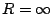.

The interval of convergence is the set offor which the series converges. It will be one of the following: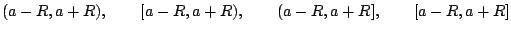The point being that the statement of the theorem only asserts something about convergence of the series on the open interval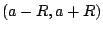. What happens at the endpoints of the interval is not specified by the theorem; you can only figure it out by looking explicitly at a given series.

Theorem 6.5.9   Ifhas radius of convergence, then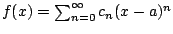is differentiable on, and
1.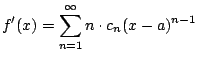2.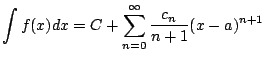,
and both the derivative and integral have the same radius of convergence as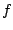.

Example 6.5.10   Find a power series representation for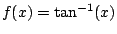. Notice that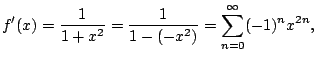which has radius of convergence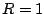, since the above series is valid when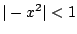, i.e.,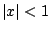. Next integrating, we find that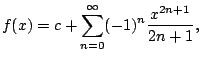for some constant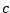. To find the constant, compute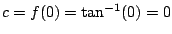. We conclude that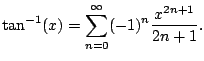Example 6.5.11   We will see later that the function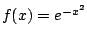has power series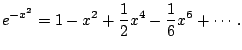Hence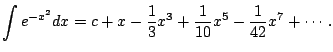This despite the fact that the antiderivative of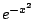is not an elementary function (see Example).

William Stein 2006-03-15